# More options for working with several buffers

For those who do not like to use the ibuffer, there is another nice feature in Emacs: Just use Ctrl+Left Mouse and you will see a buffer menu appearing which shows all the buffer modes that are active. If you click on one of them all buffers opened are shown (in the image you see the R-files opened at the moment: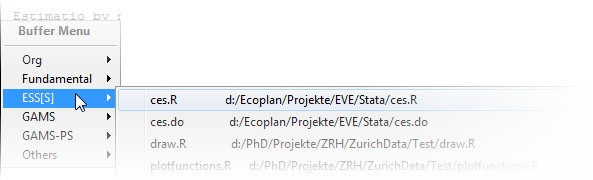Another nice key shortcut is Ctrl + Right mouse. Depending on the mode you are in it opens a menu with all the commands you can use in that mode (the next image shows you the commands that appear when you are in R (ESS-Mode)).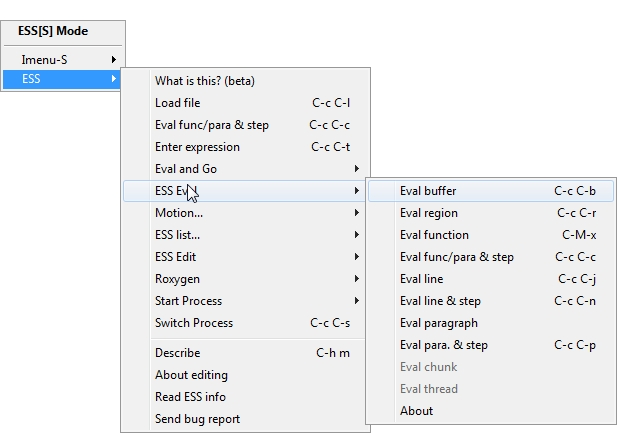# Ibuffer for looking at your buffers in Emacs

Navigating in Emacs if you have a lot of buffers open, can be done in different ways. The most basic one is using the key combination Ctrl+x Arrow Right or Left for the next or previous buffer. If there are a lot of buffers open, this is not an option. Another option is using the buffer list C-x C-b. The buffer list contains all the open buffers, but finding the one you are looking for might take some time.
I prefer to use ibuffer which offers you many possibilities for looking at your buffers. It is part of Emacs since version 22.  In the next figure you see my buffers grouped according to their mode (org, R, LaTeX, Gams, Text and all remaining buffers). The menu gives you lots of opportunities to work with the buffer list.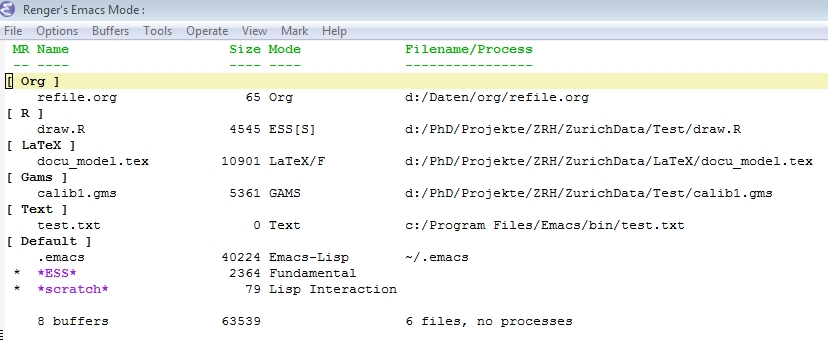One of the nice features is marking for example all org files: just put the cursor on [ Org ], hit the space bar and all files are marked (see next figure). Now you can easily save all of them or delete them. Another example: with “S” you can save all unsaved buffers.
I added the following lines to my .emacs file. The first lines set C-x C-b as the shortcut for opening the buffer list with ibuffer. The next group of commands tells ibuffer which modes to group (you can add other groups if you like).
(global-set-key (kbd “C-x C-b”) ‘ibuffer)
(autoload ‘ibuffer “ibuffer” “List buffers.” t)

(setq ibuffer-saved-filter-groups
‘((“home”
(“Org” (or (mode . org-mode)
(filename . “OrgMode”)))
(“R” (or (mode . ess-mode)
(filename . “EssMode”)))
(“LaTeX” (or (mode . latex-mode)
(filename . “LaTeXMode”)))
(“Gams” (or (mode . gams-mode)
(filename . “GamsMode”)))
(“Text” (name . “.txt”)))))

‘(lambda ()
(ibuffer-auto-mode 1)
(ibuffer-switch-to-saved-filter-groups “home”)))
(setq ibuffer-show-empty-filter-groups nil)

# Capturing todo’s and notes in Emacs

Often when I work on a model or a statistic problem in Emacs I make a note that I have to correct or add something to the code whenever I have the time for it. I used to work with Outlook or with a piece of paper, but both have disadvantages. Writing on a piece of paper or with outlook disrupts my work flow and I have to write down to which part of my work the note relates. Emacs can be a great tool for keeping track of notes or todo’s and links them directly to the file you are working on. This is done with remember-mode developed by John Wiegley
http://www.emacswiki.org/emacs/RememberMode). I use remember-mode together with org-mode (http://www.emacswiki.org/emacs/OrgMode; the manual of org-mode chapter 9 gives a good introduction in how to use remember-mode). Both modes are part of the standard Emacs version.
Let us have a look at an example. I am working on a model in Gams and know that I have to document a part of the code, but don’t want to do it right away. I hit C-c r and Emacs shows me a menu with the following options: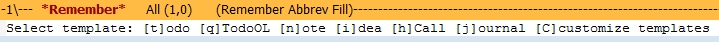I can select one of the templates I defined. I choose a “todo” which Emacs will link to the file and the place in the file I am at the moment. Emacs opens a new buffer which looks like this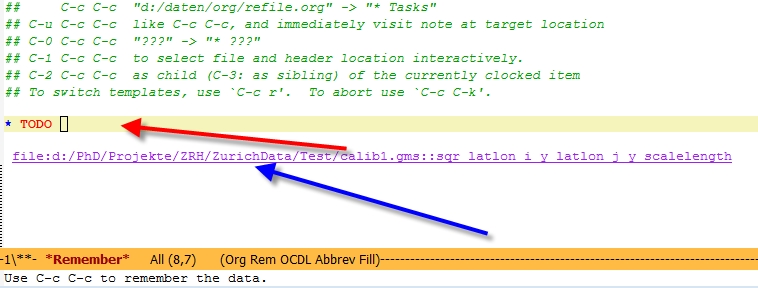I just can start typing (red arrow) and describe what I still have to do. The blue arrow is the link to the file where I am working on. I can add additional notes or tags. The green stuff gives you more information, but will not be part of the final todo. After filling out the template I hit C-c C-c and the todo is stored in the org-file “refile.org”. This is a file where all my notes, todo’s, calls etc. are temporarily stored. At the end of the day or in the morning I check this file and move the items to other files. I have for example a file phd.org with all my notes and todo’s for my phd-work.
Here is a list with todo’s in this file: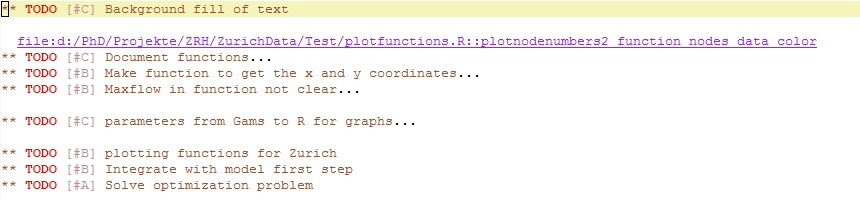The first todo is opened and you can see the link to the file I was working on when I captured this todo. Each morning when I want to work on my phd-project I scroll through my todo-list and choose the todo’s I like to work on for that day and send them to my agenda (more on that in a later post).
I have also templates for ideas, notes, calls and journal items. The set-up in my emacs file looks like this:
(define-key global-map “\C-c\ r” ‘org-remember)
(setq org-remember-templates
‘((“Todo” ?t “* TODO %?\n %i\n %a” “d:/daten/org/refile.org” “Tasks”)
(“TodoOL” ?q “* TODO %?\n %i\n ” “d:/daten/org/refile.org” “Tasks”)
(“Note” ?n “* %^{Title}\n %i\n %a” “d:/daten/org/refile.org” “New Ideas”)
(“Idea” ?i “* %^{Title}\n %i\n %a” “d:/daten/org/refile.org” “New Notes”)
(“Call” ?h “* %U %?\n\n %i  \n ” “d:/daten/org/refile.org” “Calls”)
(“Journal” ?j “* %U  %?\n\n %i\n ” “d:/daten/org/journal.org” “Journal”)))
I defined a key for capturing (C-c r) and added several templates. Take a look at the org-manual for all the options (%,? {Title}, etc.).  The tools for building templates is quite versatile and using them makes your life much easier.

# Elasticities in estimated linear models

Ever wondered how your estimation of a linear function relates to the elasticities of the estimated model? I always seem to forget, especially if I have taken the logarithm on one or both sides of the equation. Here are the four cases you can have:

Linear:
The function has the following form (if you have more variables on the right hand side, this doesn’t change the story):$Y=a + bX$

The elasticity is given by:$\epsilon= \frac{dY}{dX}\frac{X}{Y}=b\frac{X}{Y}$

and the coefficient b is the change in Y from a unit increase in X.

Log-linear$log(Y)=a + bX$

and the elasticity is given by:$\epsilon= be^{a+bX}\frac{X}{Y} = bY\frac{X}{Y} =bX$

and the coefficient b is the percentage increase in Y from a unit increase in X.

Linear-log$Y=a + b*log(X)$

and the elasticity is:$\epsilon= \frac{b}{X}\frac{X}{Y} =\frac{b}{Y}$

and b is the change in Y caused by a 1% increase in X.

Log-log$log(Y)=a + b *log(X)$

and the elasticity is:$\epsilon= \frac{bY}{X}\frac{X}{Y} =b$

Depending on your regression equation the elasticity is therefore either the estimated coefficient (double log), the coefficient multiplied divided by the left-hand variable (linear-log), multiplied by the right-hand variable (log-linear) or the fraction of right-hand and left-hand variable (linear).
By the way: the formulas were written using WordPress and the Youngwhan’s Simple Latex Plug-In for writing equations in WordPress.